# Square vs Circle (10 Interesting Things You Should Know)

Squares and circles are both important shapes in geometry, with useful applications in many disciplines.  Squares and circles have some differences, but they have some things in common as well.

So, what do you need to know about squares vs circles?  Squares & circles are both shapes with a center point. A square is a polygon with 4 sides, but a circle is not a polygon. Each shape only needs one measurement (side length/radius) to find the size (perimeter & area). We can find the dimensions of squares & circles with the same perimeter or area.

Of course, we can also examine inscribed squares inside of circles (or inscribed circles inside of squares) and relate their dimensions.

In this article, we’ll talk about how squares and circles are related in terms of their dimensions, perimeters, and areas.  We’ll also look at some examples to make the concepts clear.

Let’s get started.

## Square Vs Circle

A square and a circle are both shapes with a well-defined center.  Each shape only needs a single length to determine its size.

For a square, we need a side length to determine the size.  With this information, we can determine both the perimeter and area of a square.

For a circle, we need a radius to determine the size.  With this information, we can determine both the perimeter (circumference) and area of a circle.

A square is a type of regular polygon with four sides of equal length and four 90 degree angles.  We can also say that a square is a specific type of rectangle.

On the other hand, a circle is not a polygon (it does not have sides or angles).  We can also say that a circle is a specific type of ellipse (one where the major and minor axes have the same length, or where the foci meet to become the center).

We can also compare the perimeter and area of squares and circles.  Let’s start with perimeter.

## Square Vs Circle Perimeter

Remember that the perimeter of a shape is the path that completely surrounds the shape (no more and no less).  We can calculate perimeter as a length in linear units (such as inches, feet, centimeters, meters, etc.)

For a square with a side length of S, the perimeter is 4S.  We get this result by adding up the four equal side lengths of a square: S + S + S + S = 4S.

You can also see this from the image below.

For a circle with a radius of R, the perimeter is 2πR.  This means that we will need R pieces of string with a length of 2π to go all the way around the circle.

You can also see this from the image below.

### Square & Circle With The Same Perimeter

A square and a circle can have the same perimeter in a special case, which we can solve for below:

• Perimeter of Square = Perimeter of Circle
• 4S = 2πR
• 2S = πR

Solving for S, we get S = πR/2.  So, if we choose a radius R for a circle, we can choose a side length of S = πR/2 to get a square and a circle with the same perimeter.

Similarly, we can solve for R to get R = 2S/π.  So, if we choose a side length S for a square, we can choose a radius of R = 2S/π to get a square and a circle with the same perimeter.

Let’s see some examples.

#### Example 1: A Square & A Circle With The Same Perimeter

Let’s say we have a circle with a radius of R = 6.  For a square with the same perimeter, what would the side length be?

By the formula above, the side length is S = πR/2 = π(6)/2 = 3π (about 9.42).  We can verify as follows:

• Perimeter of Circle = 2πR = = 2π(6)= 12π
• Perimeter of Square = 4S = 4(3π) = 12π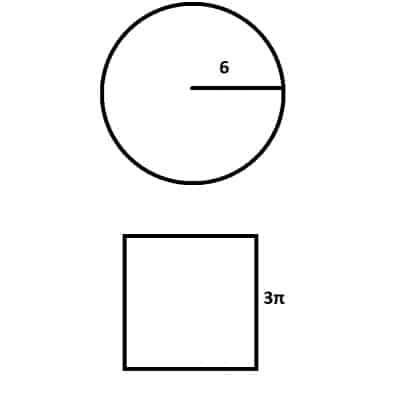A circle with a radius of 6 and a square with a side length of 3π have the same perimeter of 12π.

#### Example 2: A Square & A Circle With The Same Perimeter

Let’s say we have a square with a side length of S = 5.  For a circle with the same perimeter, what would the radius be?

By the formula above, the radius is R = 2S/π = 2(5)/π = 10/π (about 3.18).  We can verify as follows:

• Perimeter of Circle = 2πR = = 2π(10/π)= 20
• Perimeter of Square = 4S = 4(5) = 20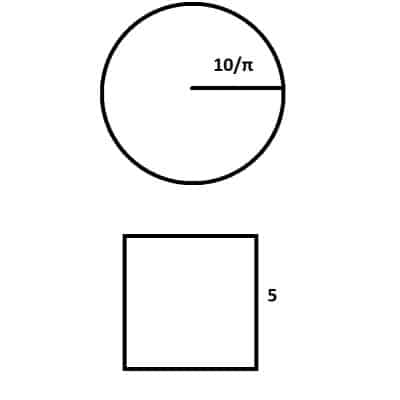A circle with a radius of 10/π and a square with a side length of 5 have the same perimeter of 20.

## Square Vs Circle Area

Remember that the area of a shape is the amount of space inside the boundary of the shape (no more and no less).  We can calculate area in square units (such as square inches, square feet, square centimeters, square meters, etc.).

These square units come from multiplying two dimensions (and thus two units) together.

For a square with a side length of S, the area is S2.  We get this result by multiplying the length by the width, or S*S, to get S2.

You can also see this from the image below.

For a circle with a radius of R, the area is πR2.  The way to see this is to break the circle into N tiny “slices” that approximate triangles (as N gets larger, the slices become closer to triangles).

The base of each slice is 2πR/N (the circumference divided into N equal parts), and the height is the radius R.  Then the area of each (approximately triangular) slice is:

• (base)*(height)/2
• (2πR/N)*(R)/2
• πR2/N

Since there are N of these slices, the area is N(πR2/N) = πR2.

You can also see this from the image below.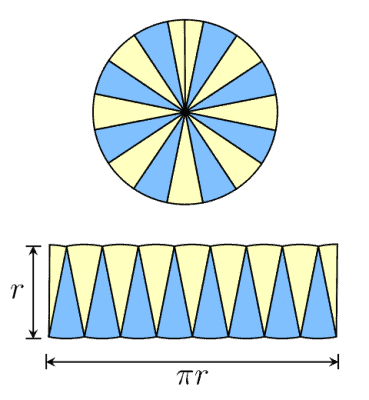If we split a circle into enough equal “slices”, they start to resemble triangles. Each triangle has a base of 2πR/N and a height of R, giving an area of πR2/N for each triangle. Since there are N triangles, the area of the circle is πR2.

### Square & Circle With The Same Area

A square and a circle can have the same area in a special case, which we can solve for below:

• Area of Square = Area of Circle
• S2 = πR2
• S = R√π

Solving for S, we get S = R√π.  So, if we choose a radius R for a circle, we can choose a side length of S = R√π to get a square and a circle with the same area.

Similarly, we can solve for R to get R = S/√π.  So, if we choose a side length S for a square, we can choose a radius of R = S/√π to get a square and a circle with the same area.

Let’s see some examples.

#### Example 1: A Square & A Circle With The Same Area

Let’s say we have a circle with a radius of R = 3.  For a square with the same area, what would the side length be?

By the formula above, the side length is S = R√π = 3√π (about 5.32).  We can verify as follows:

• Area of Circle = πR2 = π32= 9π
• Area of Square = S2 = (3√π)2 = 9π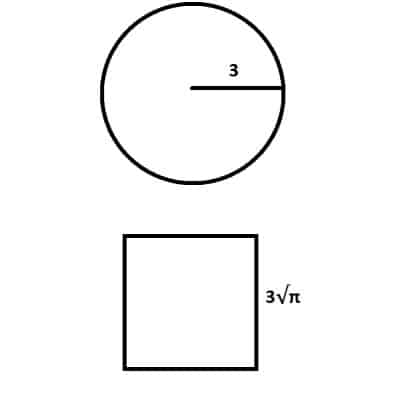A circle with a radius of 3 and a square with a side length of 3√π have the same area of 9π.

#### Example 2: A Square & A Circle With The Same Area

Let’s say we have a square with a side length of S = 4.  For a circle with the same area, what would the radius be?

By the formula above, the radius is R = S/√π = 4/√π (about 2.26).  We can verify as follows:

• Area of Circle = πR2 = π(4 /√π)2= 16
• Area of Square = S2 = 42 = 16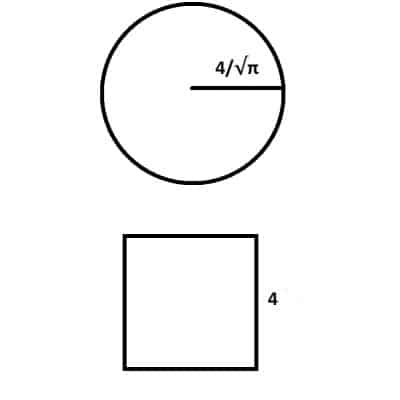A circle with a radius of 4/√π and a square with a side length of 4 have the same area of 16.

## Square In A Circle (Inscribed Square Of A Circle)

When you inscribe a square in a circle, you are finding the largest square that can fit inside of that circle.  Another way to think of it is finding the smallest circle that will contain the square.

You can see what this looks like in the diagram below.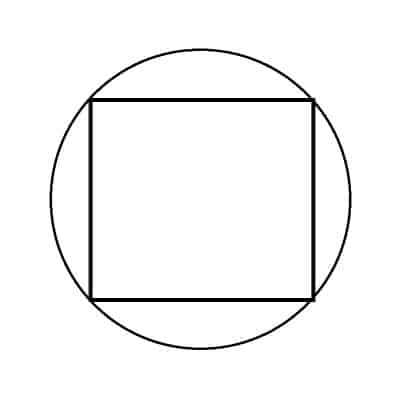Here we see the largest square that can fit inside the circle (or the smallest circle that can contain the square).

We can find the relationship between these two shapes as follows.

Let R be the radius of the circle, and S be the side length of the square.

The first step is to draw a diameter from one corner of the square to the opposite one, forming a diagonal with length R + R = 2R.  We also want to label the sides of the square as “S” (as shown in the picture below).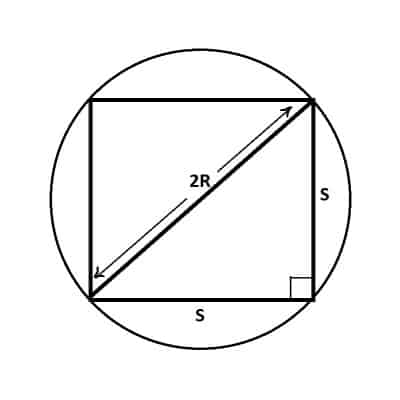The diagonal of the square is twice the radius (diameter) of the circle, or 2R. The side lengths are also labeled on the right triangle.

Now, we have a right triangle with legs S and S, with a hypotenuse of 2R.  By the Pythagorean Theorem:

• a2 + b2 = c2  [a and b are the legs, and c is the hypotenuse]
• S2 + S2 = (2R)2
• 2S2 = 4R2
• S2 = 2R2
• S = R√2

This equation tells us the relationship between the side length S and the radius R when a square of side length S is inscribed in a circle of radius R.

The side length S of the square will be about 41% larger than the radius of the circle (√2 is about 1.41).

The perimeter of the square is 4S = 4R√2 (about 5.66R).

The perimeter of the circle is 2πR (about 6.28 R).

The area of the square is S2 = (R√2)2 = 2R2.

The area of the circle is πR2.

This information is summarized in the table below.

## Circle In A Square (Inscribed Circle Of A Square)

When you inscribe a circle in a square, you are finding the largest circle that can fit inside of that square.  Another way to think of it is finding the smallest square that will contain the circle.

You can see what this looks like in the diagram below.Here we see the largest circle that can fit inside the square (or the smallest square that can contain the circle).

We can find the relationship between these two shapes as follows.

Let R be the radius of the circle, and S be the side length of the square.

The first step is to draw a diameter on the circle, from the midpoint of the left side of the square to the midpoint of right side of the square.  We also want to label the side of the square as “S” (as shown in the picture below).

Now we know that the side length S of the square is twice the radius R of the circle, or S = 2R.

The perimeter of the square is 4S = 4(2R) = 8R.

The perimeter of the circle is 2πR (about 6.28 R).

The area of the square is S2 = (2R)2 = 4R2.

The area of the circle is πR2.

This information is summarized in the table below.

## Conclusion

Now you know how squares and circles are connected in terms of perimeter and area.  You also know a little more about inscribed squares and inscribed circles.